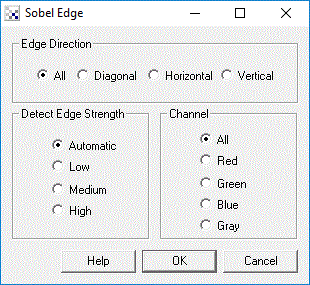# Sobel Edge

The Sobel Edge filter is use to detect edges based applying a horizontal and verticle filter in sequence. Both filters are applied to the image and summed to form the final result. The two filters are basic convolution filters of the form:

## InterfaceHorizontal FilterVerticle Filter
 1 2 1 0 0 0 -1 -2 -1
 -1 0 1 -2 0 2 -1 0 1

For example, if a 3x3 window is used as such

 p1 p2 p3 p4 p5 p6 p7 p8 p9
where the filter is centered on p5 with p4 being pixel[x-1][y] and p6 being pixel[x+1][y], etc. then the formula to calculate the resulting new p5 pixel is

new_pixel_intensity = (p1+(p2+p2)+p3-p7-(p8+p8)-p9)+(p3+(p6+p6)+p9-p1-(p4+p4)-p7)

which is them clamped to the 0-255 range.

Note that the actual formula uses the horizontal and vertical components into the final form

new_pixel_intensity = SQRT((X*X)+(Y*Y))

where X = (p1+(p2+p2)+p3-p7-(p8+p8)-p9) and Y = (p3+(p6+p6)+p9-p1-(p4+p4)-p7)

but for performance reasons we approximate the result and leave the final SQRT formula out.

Note that the new pixel values need to be placed into a new image buffer so as not to corrupt the pixel values of the current image.

For color images the formula is applied to all three color channels separately.

The Edge Strength decides how much the detected edge is reduced before converting the number back to a 8 bit (256) RGB color. Low indicates that the number is not reduced which tends to enhance lower magnitude edges, high will reduce edges such that the intensity is approximate to the image pixels. Automatic will adjust the results such that all magnitudes are scaled to the 256 range.

## Example

 Source Image Sobel